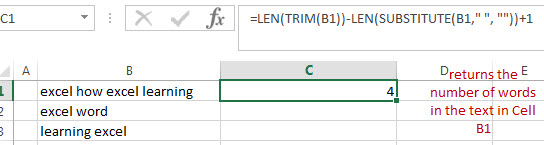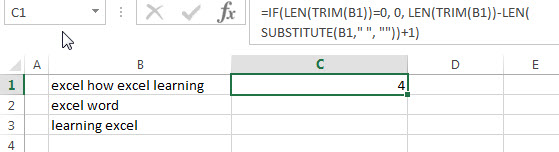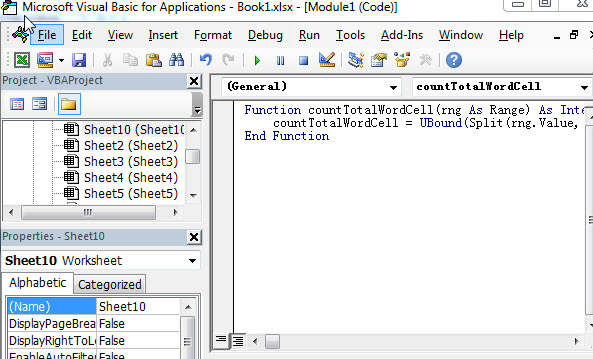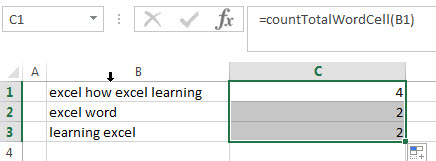# How to count the number of words in a cell in Excel

This post explains that how to count the number of words in a cell using excel formula. How to count total words in a cell with User Defined Functions in excel VBA.

## Count the number of words in a cell

If you want to count the number of words in a single cell, you can create an excel formula based on the IF function, the LEN function, the TRIM function and the SUBSTITUTE function. You need to use the SUBSTITUTE function to remove all space strings from the text string in a cell, then the returned value goes into the LEN function to get the length of the text without space string. You still need to get the length of the text with space strings and then subtract to the length of the text without space to get the number of spaces, then add 1 to get the number of words in a cell.

Assuming that you want to get the number of words in cell B1, you can write down an excel formula as follows:

`=IF(LEN(TRIM(B1))=0, 0, LEN(TRIM(B1))-LEN(SUBSTITUTE(B1," ", ""))+1)`

Let’s see how this formula works:

=LEN(TRIM(B1))-LEN(SUBSTITUTE(B1,” “, “”))+1This formula returns the number of words in the text in Cell B1. The SUBSTITUTE function replaces all space strings with empty string to remove all spaces, and using LEN function to get the length of the text without spaces.

The TRIM function will remove extra spaces from the text and just leave one space between words.

=IF(LEN(TRIM(B1))=0, 0, LEN(TRIM(B1))-LEN(SUBSTITUTE(B1,” “, “”))+1)The IF function will check if it is an empty cell, if TRUE, then returns 0, otherwise, returns the number of words in the text in cell.

## Count the number of words in a cell with User Defined Function

You can also create a new user defined function to count the number of words in a cell in Excel VBA:

1# click on “Visual Basic” command under DEVELOPER Tab.2# then the “Visual Basic Editor” window will appear.

3# click “Insert” ->”Module” to create a new module4# paste the below VBA code into the code window. Then clicking “Save” button.

```Function countTotalWordCell(rng As Range) As Integer
countTotalWordCell = UBound(Split(rng.Value, " "), 1) + 1
End Function```5# back to the current worksheet, then enter the below formula in Cell C1:### Related Formulas

• count specific words in a cell or a range
If you want to count the number of a specific word in a single cell, you need to use the SUBSTITUTE function to remove all that certain word in text string, then using LEN function to calculate the length of the substring that without that specific word.…
• Extract word that starting with a specific character
Assuming that you have a text string that contains email address in Cell B1, and if you want to extract word that begins with a specific character “@” sign, you can use a combination with the TRIM function, the LEFT function, the SUBSTITUTE function, the MID function, the FIND function, the LEN function and the REPT function to create an excel formula.…

### Related Functions

• Excel Substitute function
The Excel SUBSTITUTE function replaces a new text string for an old text string in a text string.The syntax of the SUBSTITUTE function is as below:= SUBSTITUTE  (text, old_text, new_text,[instance_num])….
• Excel IF function
The Excel IF function perform a logical test to return one value if the condition is TRUE and return another value if the condition is FALSE. The IF function is a build-in function in Microsoft Excel and it is categorized as a Logical Function.The syntax of the IF function is as below:= IF (condition, [true_value], [false_value])….
• Excel LEN function
The Excel LEN function returns the length of a text string (the number of characters in a text string).The syntax of the LEN function is as below:= LEN(text)…
• Excel TRIM function
The Excel TRIM function removes all spaces from text string except for single spaces between words. The syntax of the TRIM function is as below:=TRIM(text)…